Next: Visualizing the N-Sphere Up: The Sphere in Three Previous: The Sphere in Three

Subsections

# Overview of the Sphere

## Definitions

### Definition of the Sphere

The n-dimensional sphere is the set of all points in
space at a given radius from a center point. The radius is denoted by
and the center by
.
Unless otherwise stated, the center of the sphere is assumed to be
and the radius is assumed to be 1. Under this definition,
is the two points
in
,
is the unit circle in
, and
is the object which is commonly called the sphere or globe, in
.

### Definition of the N-Disk or N-Ball

An n-Disk is simply the set of all points inside
, or all points less than or equal tounits away from the center.
Under this definition, the 1-disk is the line segment betweenand
on, the 2-disk is the interior of the circle of radiusin, and the 3-disk or 3-ball is the interior of the sphere of radiusin
. As above, the center is assumed to beand the radius to be 1 unless otherwise specified.

### Definition of a Manifold

Throughout this paper I will use the term manifold in its standard topological sense. A n-dimensional manifold is usually defined as a set with a collection of patches, or 1-1 functions from
, whereis an open subset of
, such that the following two conditions are satisfied:

(i.) The images of the patches cover
(ii.) For two patches
and
, the composition
and
are differentiable and defined on open sets in(O'Neill).

### Definition of
, or Cartesian product

Theoperator is the Cartesian product. It takes two manifolds of dimension
and
, and duplicates the one at every point along the other, creating a manifold of dimension
.

### Definition of Generator

A generator is a manifoldcontained in another manifold
such thatcrossed with some other manifold
makes. In other words, if
, thenandare generators of. For example, since
, the generators of
are the two
.

### Definition of Equator

An equator inside
is a. This can be seen by setting one of the coordinates, say
, to zero, and taking all remaining points in.
## Construction ofby two N-disks

It's fairly trivial to see that two line segments, each end of one segment joined to an end of the other segment, make a circle (or something that can be deformed into a circle). However, the process generalizes to all dimensions, and provides us with a technique of visualizing.

Two 2-disks, or filled circles, can be joined along their circular boundaries, to make. Each disk makes a hemisphere, and the boundary between the two becomes the equator.

In general, an equator as defined above isin. Now, all the other points inare the points where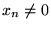. So the other points inare split into two groups:
and
. We can see that these two sets of points are N-disks because we can let
range from 1 to 0, and get a correspondingfor each value of.Next: Visualizing the N-Sphere Up: The Sphere in Three Previous: The Sphere in Three